# 包装机械,封口机械,包装封口机械,封口机械,机械,长期宏硕HS-420A点击图片查看原图 发布日期：2019-07-19 20:29 有效期至：长期有效 招商区域：广东 浏览次数：704rr
rr

r

r      r

r

r       佛山市宏硕包装机械有限公司专业生产“宏硕牌”包装机械和食品机械等产品。本公司设备齐全、技术实力雄厚、企业内部管理严密、生产效率高；本公司生产的包装机械品种多，规格齐全，并能承接各种特殊规格需求的产品定做。其机器的先进性、可靠性、稳定性已达到国际先进技术水平；产品以精良的质量、低廉的价格、美观的造型和完善的售后服务赢得了用户的信赖和好评；产品畅销全国各地，并出口东南亚、中东、欧洲等**和地区。热诚欢迎国内外各界人士来人、来电、来函洽谈惠顾，与我公司友好合作，共展宏图!r

r

r  r

r

r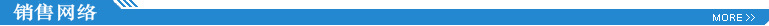r

r

r  r

r

rr

r

r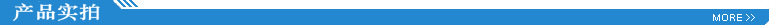r

r

r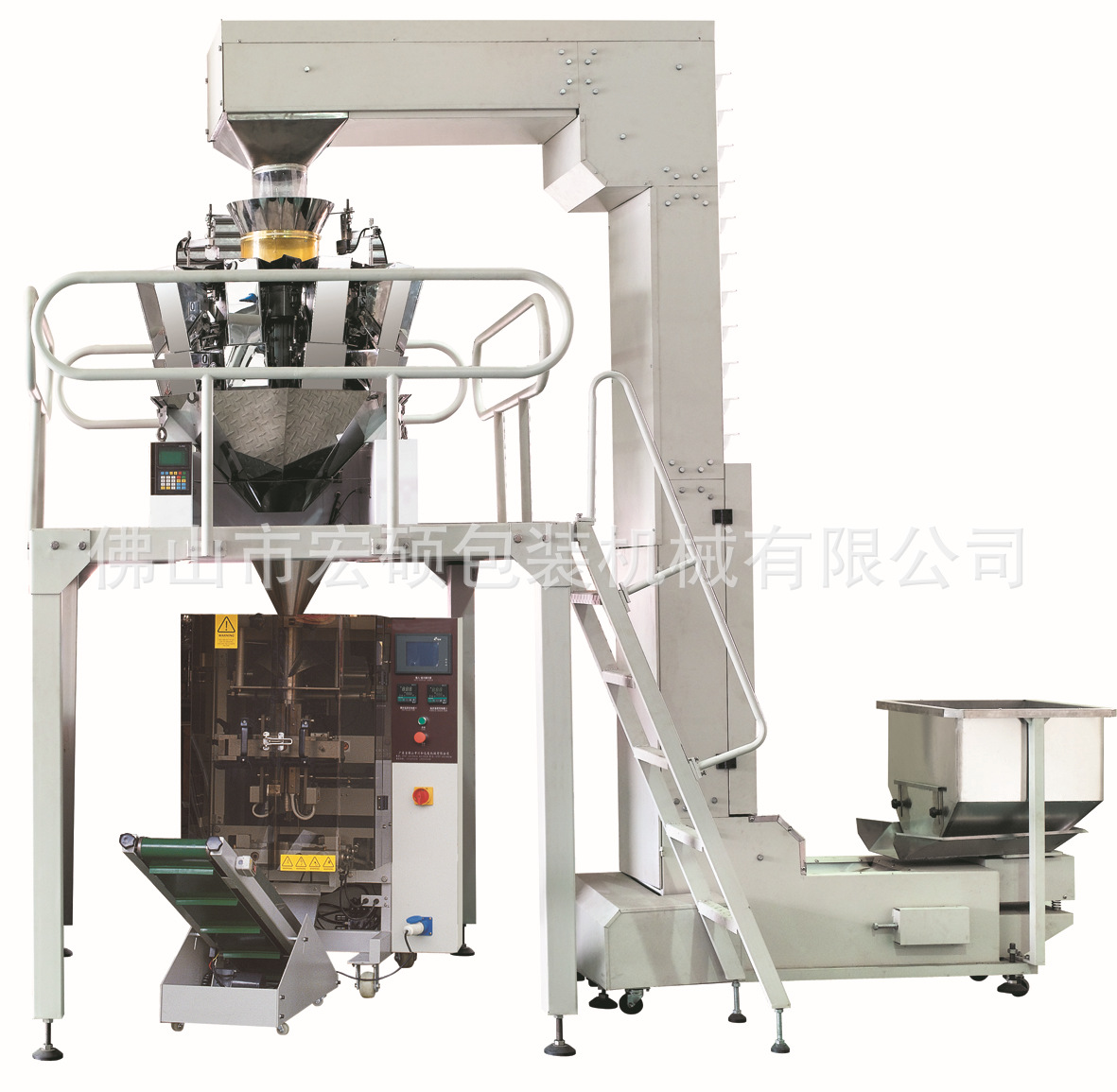r
r
r

r

r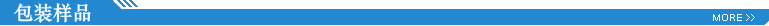r
r

r

r
r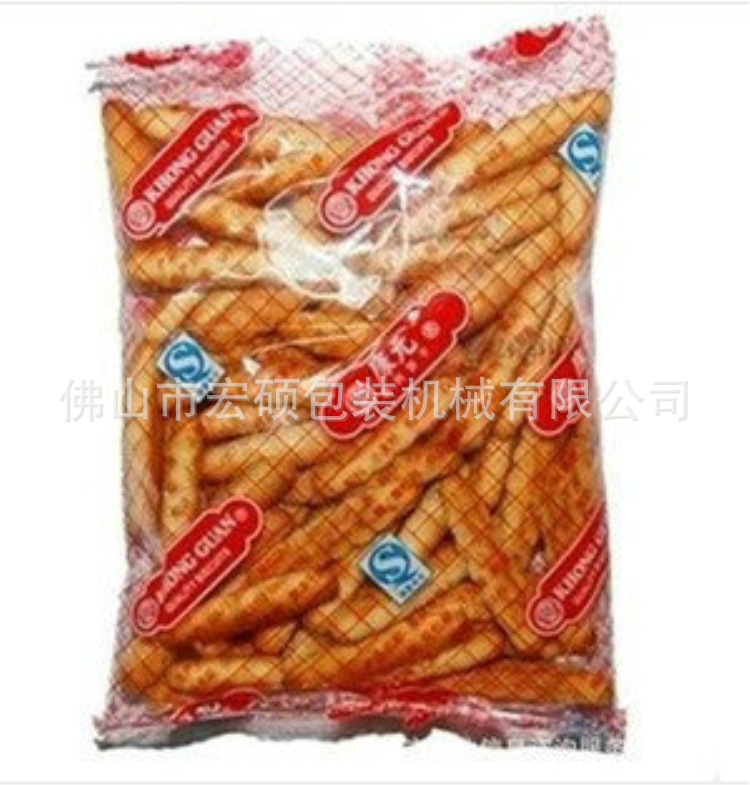r
r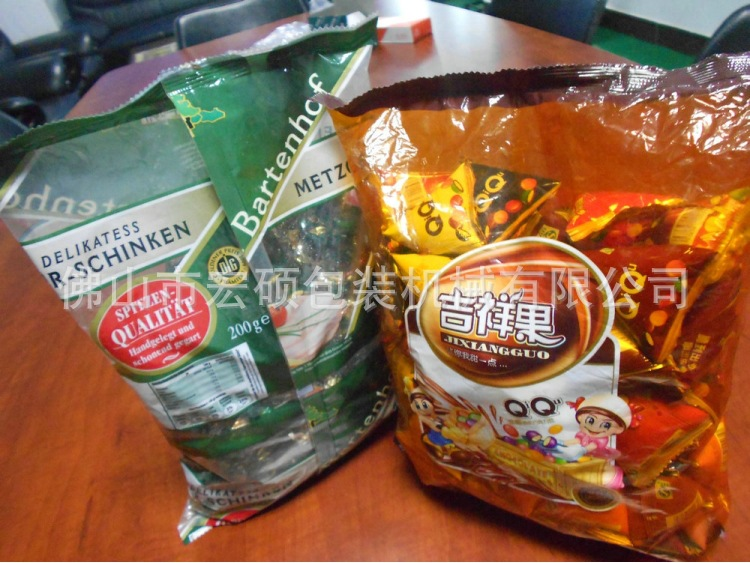r
r
【功能特点】
r一、双变频器控制，袋长即设即切，无需调节空走，一步到位，省时省膜。
r二、机械关键部位采用不锈钢结构，符合食品QS和医药GMP卫生要求。
r三、高感度电眼自动跟踪，设定后无需手动调整，封切尺寸，位置准确，封口牢固美观。
r四、温度独立PID智能控制，温控稳定，更好适合各种包装材料。
r五、故障自诊断功能，故障显示一目了然。
r六、生产自动计数，定量定产，包装产量准确了然。
r七、传动系统简洁，工作更可靠，维护保养更方便。
r八、包装产品物料位置参数化设置，无需手动机械式调节包装料位。
r九、可储存数组包装产品的参数设置，更换包装产品时，直接切换即可正常生产，无需重新调整机械。更换包装产品，省时省膜。r

r

r 十、智能人性化参数设计，普通人员短时间内能熟练使用。降低对操作人员的专业技能求。
r十一、本机型可根据用户需要配置单伺服、双伺服、全伺服。r

r

r  r

r

r
r

r

r 【适用范围】
r适合于食品、药品、日用品、五金零件、数码配件、或带托盘的各类有规则物体的包装。r

r

r 如：食品  (饼干、糖果、面条、蛋黄派、月饼、方便面、冰棒、饺子、速冻食品...)
r      药品   （胶囊、药品、眼药水、口罩...）
r      日用品（一次性用品、湿巾、百洁布、玩具、双面胶、酒店洗漱用品...）
r      工艺品（小工艺品、树脂工艺品...）
r      五金零件  (铰链、导轨、合页、皮带扣、 拉手、轴承、点火器 ...)
r      数码配件  (手机壳、数据线、充电器...)r

r

r  r

r

r
r

r

r 【技术参数】r

r

r  ...r

r

r  r

r

r （三）、整套机组技术参数：r

rr r r r r r r r r r r r r r r r r r r r r r r r r r r r r r r r r r r r r r r
 r r 项    目 Item          r r r r  内  容 Contentr r r r 包装速度 r r r Capacityr r r r 约60包/分钟 具体视包装膜质量及物料确定。r r r 45bag/min,it is according to quality of wrapping and suppliesr r r r 计量精度r r r Accuracyr r r r ≤±1.5% r r r r 制袋尺寸  r r r Bag sizer r r r (L)60_300mm (W)60_200mm r r r r **大包装膜宽r r r Film widthr r r r 420mm r r r r 制袋形式r r r Bag typer r r r 包装材料用卷膜，机器自动制袋为上封、下封加背面封口。r r r Pack with film, upper seal, lower seal and back seal by automatic bag making machine. r r r r 薄膜厚度r r r Film thicknessr r r r 0.04-0.09mm r r r r 包装材料r r r Package materialr r r r BOPP/CPP，PET/AL/PE等可热复合材料r r r thermal composite material., likeBOPP/CPP, PET/AL/PE etcr r r r 整套耗气量  r r r Air consumption   r r r r 0.8Mpa 0.4m3/minr r
r

r ........................................................................................................................................................................................r

r

r 【可选配置】
r1、充气装置   2、连包装置    3、特殊的封口方式      4、全不锈钢 r

r

r 5、打码机       6、排气装置   7、防空包防切料装置r

r

r  r

r

r
r

r

r 【采购要项】
r一.【生产周期】一台7个工作日内，两台以上以实际情况而定。r

r

r
r

r

r 二.【运输方式】 □ 自提   □铁路运输   □ 空运   □ 汽运r

r

r
r

r

r 三.【付款方式】30%定金，设备完成生产后付清余款。r

r

r
r

r

r 四.【随机配件】内六角扳手1套、活动扳手1把、十字一字螺丝刀各一把、发热管中封、端封各两条、切刀一对、中封皮带一条。r

r

r
r

r

r 五.【质量保证】 国内：一年内非人为所造成的机械故障，我公司一律实行三包。超规定范围，我司实行标准收费； 设备终生维护。r

r

r 国外：一年内非人为损坏的配件免费更换，邮费买家负责，我司提供终身技术支持。r

r

r
r

r

r 六.【注意】由于包装机多数属于非标产品，具体的价格以实际定做规格为准，买家可来电或通过网络和我取得联系，以**商谈结果为准。r

0相关评论